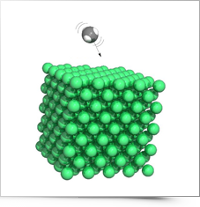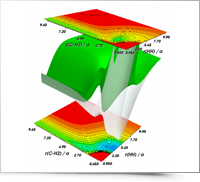Top

## teaching

### Physical Chemistry B (Master Degree in Chemistry)Prof. Rocco Martinazzo

ECTS credits: 6, Code: F5Y-9

Simple models of metals
Drude model: DC and AC conductivty, magnetotransport and Hall effect, thermal conductivity, thermopower. Sommerfield model: Fermi sphere, density of states; energy, pressure and entropy of the free-electron quantum gas. Fermi-Dirac statistics: gran canonical parition function, Fermi function, Sommerfield expansion.
Crystal structure
Lattices, basis vectors and unit cells. Bravais lattices. Reciprocal lattice. Lattice planes, Miller indices. Investigation of crystal structures: Thomson diffusion, X ray diffraction, von Laue vs. Bragg laws, structure factor.
Electrons in periodic potentials
Bloch theorem: Bloch functions, energy bands. Tigh-binding method and application to model systems: linear chains, two- and three-dimensional materials. Peirls distortion. Graphene electronic structure: Dirac cones, chiral fermions.
Semiclassical electron dynamics
Wavapackects, uncertainty principle, semiclassical equation of motion, phase-space distribution function. Fundamental properties: inertness of filled bands, electrons and holes, effective mass tensor. Drude-Boltzmann theory of DC and AC charge transport. Optical conductivity. Diffusive vs. balistic motion. Disordered systems.
Semiconductors
Number density of charge carriers in thermal equilibrium, mass law, chemical potential. Electron and hole doping. Heterogeneous semiconductors: p-n junction, potential profile, current. Interfaces: metal/semiconductor, semiconductor heterostructures, quantum wells. Delta doping and remote doping. Two-dimensional electron-gases and electrostatic gating. Surfaces and Fermi level pinning.
Phonons
Normal modes, statistical mechanics of harmonic oscillators. Phonon dispersion curves, acoustic and optical phonons. Phonon heat capacitances, Einstein and Debye models. Lattice thermal conductivity, phonon-phonon scattering.
Reference books
• N. W. Ashcroft and D. N. Mermim, Solid State Physics, Saunders College Publishing
• T. Ihn, Semiconductor Nanostructures, Oxford University Press

### Physical Chemistry III (1st level Degree in Chemistry)Prof. Rocco Martinazzo

ECTS credits: 6, Code: F5X-98

Statistical Thermodynamics. The concepts
The need of a statistical description. Boltzmann distribution and partition function. Canonical ensemble, configurations and weights. Basic properties. Molecular Partition Function. Mechanical variables, internal energy and generalized forces. Simple examples. Entropy, temperature, Helmholtz free energy. Thermodynamic potentials. Gibbs energy and Gibbs-Duhem equation.
Statistical Thermodynamics. Applications
Statistical mechanics of molecules: translational, rotational, vibrational and electronic contributions to the molecular partition function. Mean energies, heat capacities, entropy. Real gases: configurational integral, virial expansion, van der Waals equation. Chemical equilibrium.
Solid Materials
Lattices, basis vectors and unit cells. Bravais lattices. Reciprocal lattice, lattice planes, Miller indices. Scanning Tunneling Microscopy, Atomic Force Microscopy, Transmission Electron Microscopy. X-ray diffraction: interference, diffraction, von Laue condition, structure factor. Bonding and packing in solids: metallic, ionic, covalent and molecular solids. 2D and 1D materials. Electronic structure of solids: band theory, group velocity, electron transport. Metals, semiconductors and insulators. Doping in semiconductors, p-n junction. Optical properties: Drude's and Lorentz models, dielectric permittivity, wave propagation. Excitons and polarons in condensed phases.
Molecules in Motion
Kinetic model of gases: Maxwell distribution, collision frequency, mean free path. Collisions with surfaces. Rate of diffusion. Transport properties of a perfect gas.
Reaction Dynamics
Collision theory: collision rates in gases, cross–section, energy and steric requirements, RRKM model. Diffusion controlled reactions. Transition State Theory: Activated Complex, Eyring equation. Thermodynamics aspects. Dynamics of molecular collisions: reactive collisions, potential energy surfaces, experimental and theoretical results.
Reference books
• P.W:Atkins, Physical Chemistry, Oxford University Press

### Theoretical Chemistry (2nd level Degree in Chemistry)Prof. Rocco Martinazzo, Prof. Michele Ceotto

ECTS Credits: 6, Code: F5Y-1

Introduction
Linear algebra. Dirac notation. Time-dependent Schrodinger equation (TDSE). Variational principles and perturbation theory.
Separation of electronic and nuclear motions
Born–Oppenheimer approximation. Adiabatic and diabatic states.
Wavefunction methods for electrons
The N–electron problem. Orbitals and Slater determinants. Basis functions. Hartree-Fock approximation. Electron correlation: configuration interaction and perturbative approaches.
Density Functional Theory for electrons
Hohenberg–Kohn theorems. Kohn–Sham equations. Density functionals. Pseudopotentials. Applications.
Chemical reaction theory
Collision theory in classical and quantum mechanics. Scattering operators. Numerical solution of the TDSE. Feynman's path integrals. Semiclassical theory. Transition state theory. Brownian motion and Langevin equation. Kramers theory.
Reference Books
• A. Szabo and N.S. Ostlund, Modern Theoretical Chemistry, Mc Graw-Hill Inc., New York, 1989
• R. G. Parr and Yang, Density–Functional Theory of Atoms and Molecules, Oxford University Press, New York, 1989
• D. Tannor, Introduction to Quantum Mechanics: A Time–Dependent Perspective, University Science Books, Sausalito, CA, 2007